﻿ The Limitations of Campus Wireless Networks: A Case Study of University of Nigeria, Nsukka[Lionet] Scientific & Academic Publishing: The article detailed informationInternational Journal of Networks and Communications

p-ISSN: 2168-4936    e-ISSN: 2168-4944

2012;  2(5): 112-122

doi: 10.5923/j.ijnc.20120205.04

### The Limitations of Campus Wireless Networks: A Case Study of University of Nigeria, Nsukka[Lionet]

Udora N. Nwawelu , Anthony N. Nzeako , Mamilus A. Ahaneku

Department of Electronic Engineering, University of Nigeria, Nsukka, Enugu, Nigeria

Correspondence to: Udora N. Nwawelu , Department of Electronic Engineering, University of Nigeria, Nsukka, Enugu, Nigeria.
 Email: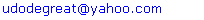Abstract

This work studied the effects of physical environment (trees and buildings) obstructions on the Lionet in Nsukka campus of the University of Nigeria. The effects of these obstructions were measured by the variation in path loss and received signal strength as functions of distance using computer simulation and field measurement. The simulation results were compared with the field measurement results, conducted under free space condition and where the Lionet signal was obstructed by trees and buildings. The results show that trees and buildings have significant effects on the path loss and the received signal strength of the Lionet. The study therefore recommends that the power level of the Lionet should be improved for efficient performance, more access points should be deployed at locations where obstructions are prevalent, and Lionet should possibly migrate from wireless to fibre optic network.

Keywords: Physical environment, Received Signal Strength, Path Loss, Free Space

### 1. Introductio

Wireless communication system refers to any system that uses electromagnetic waves to transfer information from one point to another without using wires. In communication systems where antennas are used to transmit information from one point to another, the medium between (and around) the transmitter and receiver has a major influence on the quality of the transmitted signal. This is because radio wave propagation is very sensitive to the properties and effects of the medium located between the transmitting and the receiving antennas. When radio wave impinge on an obstruction, some of the energy can be reflected back into the initial medium, some may be transmitted into the second medium, some may be absorbed by the medium, some may be diffracted and some may be scattered. The interaction of radio waves with the obstruction reduces its received signal strength.
The University of Nigeria Nsukka, in Enugu State of Nigeria, operates wireless network communication system for its diverse activities. Many ornamental trees for aesthetic and large buildings constitute major physical features of the University environment. For the fact that radio waves are very sensitive to obstructions, the trees and buildings are expected to have significant effects on the received signal strength, besides other attenuating factors such as the terrain. This factor ought to be taken into consideration in the deployment of wireless communication networks in the University environment. The University has land mass of about 871.38 hectares in an asymmetrical shape lying to the Northeast of Nsukka town. The bulk of the buildings in the University occupy the Western half of the site. Buildings are known to be main source of signal attenuation, but vegetation elements such as trees also have some reducing effects on a propagated radio signal. When the radio waves are being transmitted through the buildings, the penetration loss causes the signal to be severely attenuated. For the trees, the discrete scatterers such as the randomly distributed leaves, twigs, branches and tree trunks can also cause attenuation, scattering, diffraction, and absorption of the transmitted radio signal.
The poor signal reception frequently experience in the University has been attributed to the obstruction of the Lionet signal by the trees, buildings e.t.c. For the buildings, scattering, absorption and diffraction of the Lionet signal when it interacts with the buildings could be the main cause of signal degradation and fluctuation. For the case of the trees, Lionet signal propagating through them is attenuated because of the absorption of power by the components of the tree canopy such as leaves, branches e.t.c. There are also some losses in the transmitted Lionet signal due to scattering of power out of the beam by the components of the tree canopy. Preliminary investigations around the Lionet access points revealed that the subscribers usually receive the signal better around the trees nearer to access points. This is evidence that the propagated Lionet signal is being obstructed by trees and buildings, thus preventing it from covering its maximum expected range.
Studies on the effects of vegetation and buildings on the transmitted radio signal have been done. Propagation measurements through vegetation over a range of frequencies have been carried out in rain forests of India between 50 – 800 MHz, through a single tree between 1 and 4 GHz, in a forested urban park between 0.9 and 1.8 GHz, in various foliage and weather condition between 2 and 60 GHz. The effects of vegetation on GSM signal propagation in rural areas has been studied. Also, a good number of research works on data acquisition were reviewed. These dealt with measurements to study the effects of a single tree, a line or multiple lines of tress along the streets or buildings, a small forest, and a large forest covering a region on the propagated radio signal15]. Investigation of foliage effects on a propagated radio signal via data logging for point-to-point link at 5.8 GHz was reviewed. The result showed that point-to-point link which was obstructed by a single tree and a row of trees recorded an average of 12.2615 dB and 4.6306 dB excess path losses respectively. In, study on the influence of trees on radio channel at frequencies of 3 and 5 GHz shows that the excess path loss was between 1 and 16 dB. For the measurements carried out in the residential areas and homes for the transmission frequency in the 5.85 GHz band, building penetration attenuation was reported at an average of 14 dB.
The present study is motivated by the undesirable poor signal reception, loss of data packets, and delay in uploading and downloading of data, frequently experience from the radio wave signal transmitted by the Lionet within the University environment. The study investigates the extent of signal attenuation due to the trees and buildings, by using spectrum analyzer (SPECTRAN HF 6080) to monitor the mean path loss and received signal strength from one of the Lionet’s signal access point in the University. The modeling equations describing the signal attenuation due to tree and building obstructions are developed for detail analysis and in-depth understanding of the scenario. A computer simulation programs based on MATLAB is used to study the variations in the path loss and received signal strength as functions of the separation distance between transmitting and receiving antennas to validate the results from the spectrum analyzer.

### 2. Suggested Tree Path Loss Propagation Model

Path loss prediction of Lionet signal passing through a tree includes; free space loss plus tree loss factor (Ltree). The tree loss factor is included in the model to account for the increase in attenuation of the received signal strength when the receiver is placed behind a tree. Mathematically, this becomes.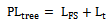(1)
PLtree = Path loss in the presence of a tree
LFS = Free space loss
Lt = Tree loss factor
The geometry of the propagation model is shown in figure 1. As illustrated in the figure, the access point radiates a spherical wave that can reach a client behind a tree, r is the separation distance between the transmitting and the receiving antenna, Pi corresponds to the power emitted (incident power) from the transmitting antenna, Pr is the received signal strength (received power level) by the receiving antenna, Ps and Pa correspond to the power scattered and power absorbed by the elements of the tree canopy.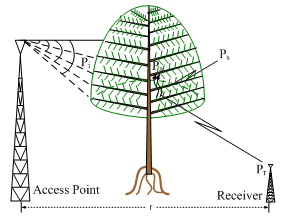Figure 1. Geometry of tree Canopy
A tree canopy contains randomly distributed and oriented branches and leaves that fill most of the canopy space. In this study, branches are modeled as circular cylinders while leaves are modeled as thin disks as described in. The branches in the tree canopy are classified into five size categories denoted as Nb. The number of leaves and branches are represented by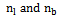respectively, where the subscript b is an element of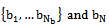is used to denote branches of the nth size category.
Attenuation of radio wave propagating through a tree is primarily due to scattering and absorption caused by both leaves and branches.
Some assumptions made while trying to reduce the complexity of the model include:
• Loss due to the trunk as a result of diffraction is considered negligible.
• The tree is so tall that there is no diffraction.
• The leaves are assumed to be identical and homogenous.
• Multiple scattering is negligible.
• The tapered nature of the individual branches is neglected.
From figure 1, the power balance in the volume is;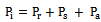(2)
For a sphere of radius r with center at the ray intersection, the beam subtends an area A on the sphere surface. The solid angle is given by;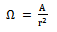(3)
The infinitesimal element of solid angle can also be written in terms of elementary area dA on any surface. The element of solid angle is;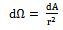(4)
The incident power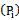is readily related to power density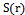, the magnitude of the time-average pointing vector as;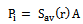(5)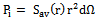(6)
The received power level can be written as;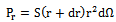(7)
As the incident power travels downwards in the tree canopy, it undergoes both absorption and scattering by the leaves and branches of the canopy. The power loss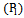contributed by the scattering and absorption of incident power by the leaves, represented by their number n1 and branches classified into Nb different size categories represented by their number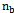is: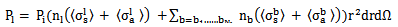(8)
For brevity,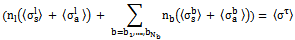Therefore equation (8) becomes;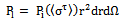(9)
Superscript b and l refer to leaves and branches of the canopy
Substituting equation (6), (7) and (8) into equation (2) we have;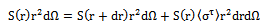(10)
This simplifies as;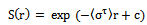(11)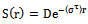(12)
where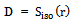is the emitted power density in a spherical wave generated by an isotropic antenna with a time-average transmitted power Pt,.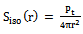(13)
Substituting equation (13) into equation (12) we have;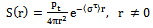(14)
For a more realistic antenna, the radio wave is not only transmitted in the desirable direction but also in other less desirable direction. A commonly used parameter to measure the overall ability of an antenna to direct radiated power in a given direction is their directive gain.
A desirable property during transmission between two antennas is that the ratio between the transmitted and received power should be as high as possible. Thus;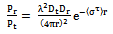(15)
Where;
Dt = the directivity gain of the transmitting antenna.
Dr = the directivity gain of the receiving antenna.
λ = the wavelength.
The equation (15) can only be employed when the leaves and branches occupied all spaces between the transmitting and receiving antenna. To get a more realistic expression for the attenuation of the emitted radio wave, we considered a case whereby the radio signal traversed estimated number of leaves and branches before getting to a receiver. The improved formula of equation (15) becomes;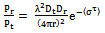(16)
Transforming equation (16) into decibels and expressing it in the path loss form yields;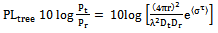(17)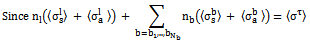Let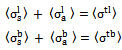Hence, equation (17) becomes;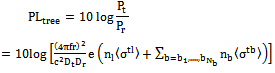(18)
where;
f = Frequency of transmission (MHz)
c = Velocity of light in air = 3*108 (m/s)
= Branches of different size categories
= Number of leaves of the tree canopy
= Number of branches of the tree canop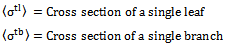#### 2.1. Expectation Value of the Total Cross Section

The total cross section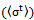is the sum of the cross section due to absorption and scattering of incident power density by a single leaf and a single branch. It is written as: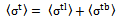(19)
The value of total cross section depends on canopy attributes such as size, shape, dielectric properties of leaves and branches, and orientation of leaves and branches in relation to the direction of the propagation of radio wave. Here, we assumed that the total cross section is a function of orientation angles, the number of leaves and branches, the size of the leaves and branches, and the shape of the leaves and branches. Figure 2 shows the interaction of incident power with a single leaf or a single branch.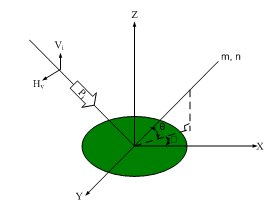Figure 2. Incident Wave on a Single Leaf or a Single Branch of the Tree Canopy
The orientation of leaves and branches determine how radio wave is being intercepted and absorbed as it traverses the tree canopy.
For the orientation angle of the leaves and branches with respect to the reference frame, we used two variables (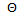,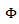) to describe it.
where;
= Elevation orientation angle of leaves and branches of the tree canopy.= Azimuthal orientation angle of leaves and branches of the tree canopy.
The normal of the disc is denoted by n and the symmetry axis of the cylinder by m. From figure 2, the normal vector which also is valid for the symmetry axis of the cylinder can be written as;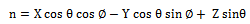(20)

#### 2.2. The Expectation Value of the Total Cross Section

The general expression for the expectation value of total cross section of a single leaf and a single branch of the tree canopy is;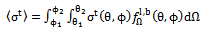(21)
where dΩ = cosθ dθ dis the solid angle,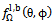equals the probability density function for the azimuthal and elevation orientation angle of the leaves and branches of the tree canopy.
Based on the fact that leaves and branches in the canopy are randomly placed, we employed a statistical based approach to characterize the leaves and branches of the canopy. This relation takes the form of probability density function. The probability density for the leaves and branches in the azimuthal coordinateis assumed to be uniformly distributed over the tree canopy volume from 0 ≤< 2π. Also, the probability density for the leaves and branches in elevation coordinateis considered to be uniformly distributed and of the form;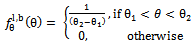(22)
Elevation and azimuthal orientation angle of leaves in the tree canopy: None of the leaves in the tree canopy were found to exhibit a preferred azimuthal orientation angle; also the leaves have no preferred elevation orientation angle. Thus, the cross sections of the leaves are averaged overandwith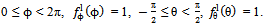. The mean cross section of a single leaf of a tree canopy is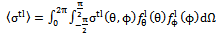(23)
Elevation and azimuthal orientation angle of the branches in the tree canopy: None of the branches of the tree were found to exhibit a preferred azimuthal orientation angle; also the branches have no preferred elevation orientation angle. Thus, the cross-sections of the branches are averaged over ϕ andwith 0 ≤ ϕ < 2π,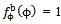,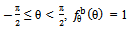. The mean cross section of a single branch of a tree canopy is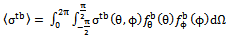(24)
By combining equation (23) and (24) for the case of mean cross section of a single leaf and a single branch of a tree canopy; we obtain an expression for the equivalent cross section of the leaves and branches of the tree canopy as;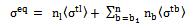(25)
As previously stated, the cross section of a single leaf and a single branch are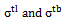; the number of leaves and branches of a tree canopy are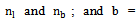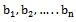is the number of branches of different size categories.
 Table 1. The Number of Leaves and Branches per Unit Volume of a Tree Canopy
 Scatterer Type Radius (m) Length/thickness (m) Number Branch 1 = b1 0.057 1.1000 3 Branch 2 = b2 0.047 1.0000 6 Branch 3 = b3 0.031 0.7200 8 Branch 4 = b4 0.022 0.5200 11 Branch 5 = b5 0.008 0.1100 15 leaf 0.063 0.0002 200
In order to get an estimated value of the number of leaves and branches per unit volume of the tree canopy, we counted the number of branches grouped into five categories and the number of leaves that are attached to the respective branch. The estimated number of leaves and branches with their corresponding radius and length/thickness per unit volume of the tree canopy is listed in the table 1.

### 3. Suggested Building Path Loss Propagation Model

Path loss prediction of the Lionet signal passing through a building includes; free space loss plus building loss factor. The building loss factor is included in the model to account for the increase in attenuation of the received signal strength when the receiver is placed behind a building. The expression for the path loss from the transmitting antenna to the receiving antenna in the presence of a building is;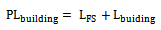(26)
where;
PLbuilding = Path loss in the presence of building.
LFS = Free space loss.
Lbuilding = Building loss factor.
The geometry of the propagation model is shown in figure 3.
Modeling Lionet signal transmitted through a building involves three steps; the first step relating the output of the Lionet signal from the transmitter to the building, the second step is called an indoor event, and the third step relating the propagation of the signal down to the receiver antenna.
As illustrated in the figure 3, a transmitting antenna radiates rays that can reach a receiving antenna.
Ht = the transmitting antenna height
Hr = the receiving antenna height
wb = the width of the building
d1 = the distance from the transmitting antenna to the building
d2 = the distance from the building to the receiving antenna
t = the window and wall thickness.
dt = the path length from the transmitting antenna to the building in the direction of signal propagation.
din = the path length inside the building in the direction of signal propagation.
dr = the path length from the building to the receiving antenna in the direction of signal propagation.
θ = the incidence angle on the window and wall of the building.Figure 3. Geometry of Lionet Signal Transmission through a Building
Some assumptions made while trying to reduce the complexity of the model include:
• The access point is assumed to be in a transmitting mode, while the receiver is assumed to be in the receiving mode.
• The length of the building is assumed to be infinitely large, so no diffraction effects could happen due to the corners of the building.
• The building window and wall are made of certain kind of material, which had relative permittivity εr and conductivity σ.
• The height of the building is so high compared to the transmit antenna height, so no propagation over the roof exists.
• The Lionet signal comes from outside the building, penetrates into it through a window and wall. Likewise the signal going from inside the building to outside.
• The direction of signal propagation does not change for the signal moving inside the building.
• The internal layout of the building was not specified.

#### 3.1. Free Space Loss Model

The equation for free-space propagation between two antennas is given by the Friis transmission equation;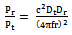(27)
where;
Pt = the transmitted power
Pr = the received power level
Dt = transmit antenna directivity gain
Dr = receive antenna directivity gain
r = the transmit and receive antenna distance of separation
c = the velocity of light
f = the transmission frequency

#### 3.2. Building Loss Factor

When the Lionet signal passed from the building to the receiving antenna, an expression for the building loss factor is written as: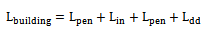(28)
where;
Lpen = Penetration losses
Lin = Indoor losses
Ldd = Distance dependent losses
Here, we considered only the signal that passed through the window and wall. For the two scenarios, their signal strengths are summed up. The angle that the Lionet signal made with the window and wall is obtained from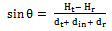(29)
From equation (29), expression for the elevation angle is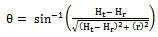(30)
Once the elevation angle is known, the expressions that relate the distances dt, din, and dr with θ are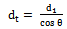(31)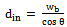(32)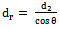(33)
Penetration Losses
Losses as a result of Lionet signal coming from outside to the inside of a building are group into two; window penetration loss and wall penetration loss. These losses were modeled using Fresnel Transmission Coefficient (FTC). This parameter characterizes the level of signal strength coming from outside into the building. The expression for the Fresnel Transmission Coefficient was given as;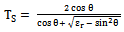(34)
By normalization, the dependence of penetration losses with the Fresnel Transmission Coefficient is;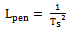(35)
The penetration losses are the same both when the signal is coming from outside the building, and when is coming from inside to outside of the building due to reciprocity law.
We assumed that the Lionet signal traversed two windows and two walls before getting to a receiving antenna. We present below a method of obtaining the window penetration loss and wall penetration loss.
Window Penetration Loss
The Lionet signal penetrates two windows made of glass before getting to the receiving antenna positioned at a distance away from the transmitting antenna. The window penetration is illustrated in Figure 4.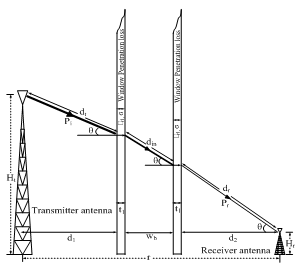Figure 4. Configuration for Lionet Signal Penetrating the Glass Window
Table 2 shows the parameters and formulas employed for predicting the window penetration loss.
 Table 2. Window Penetration Loss Parameters and Formulas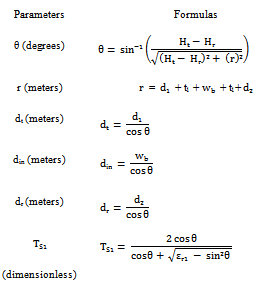From the information given in the table 2, loss as a result of the penetration of the Lionet signal through a glass window is given by;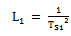(36)
where;
L1 = the window penetration loss
εr1 = the relative permittivity of the window made of glass
Ts1= the Fresnel Transmission Coefficient of the window
Wall Penetration Loss
The Lionet signal penetrates two walls made of concrete before getting to the receiving antenna positioned at a distance away from the transmitting antenna. The wall penetration is illustrated in Figure 5.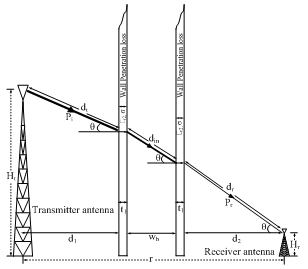Figure 5. Configuration for Lionet Signal Penetrating the Wall
Table 3 shows the parameters and formulas employed for predicting the wall penetration loss.
 Table 3. Wall Penetration Loss Parameters and Formulas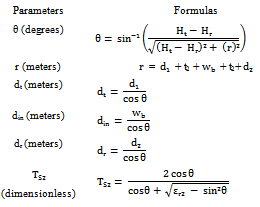From the information given in the table 3, loss as a result of the penetration of the Lionet signal through a wall made of concrete is;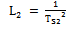(37)
Where;
L2 = the wall penetration loss
εr2 = the relative permittivity of the wall made of concrete
TS2 = the Fresnel Transmission Coefficient of the wall
In order to evaluate the Fresnel Transmission Coefficient, which measures the quantity of the building penetration loss, the relativity permittivity of the materials of interest is considered. Suitable values were gotten from literature. For glass, its relative permittivity has been found to be 4. Therefore, the relative permittivity of the window made of glass (εr1) is assumed as 4. For the wall made of concrete, the relative permittivity at 1.7 GHz and 18 GHz are 4.62 and 4.11 respectively. From these results, it is obvious that the relative permittivity of the concrete wall does not vary significantly due to frequency changes, therefore the relative permittivity of the concrete wall (εr2) is assumed as 4.62.

#### 3.3. Distance Dependence Losses

Distance dependence losses are dependent on the distance from the building to the receiving antenna. The path loss dependence with distance from the building to the receiver was chosen equal to 2 as in the case of free-space loss because it obeys power decays law. The resultant expression is;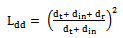(38)
where;
dr = path length from the building to the receiving antenna in the direction of signal propagation.
By combining equations (27), (36), (37), (38) an expression to predict the path loss that the Lionet signal suffered when transmitted through the building becomes;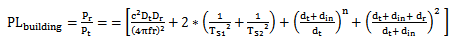(39)
n = Path loss exponent value for the 'same floor' measurement.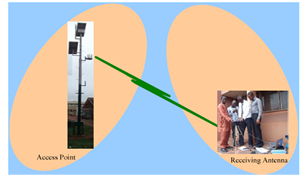Figure 6. Free Space Condition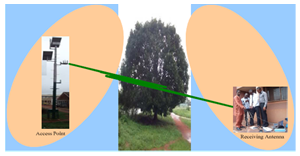Figure 7. Obstruction of Lionet Signal by a Single Tree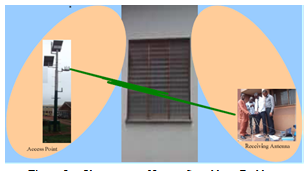Figure 8. Obstruction of Lionet Signal by a Building

### 4. Experimental Setup and Measurements

The experimental setup for measuring the path loss and received power level under free space condition and where a single tree or a building obstructs the Lionet signal are shown in figures 6, 7, and 8, respectively. At the transmitting section, omni-directional antenna (WBS 2400) with transmitting power of 19 dB is mounted at the top of 7m height mast Ht. Along the transmission path lays either a tree or a building, which obstructs the Lionet signal as in the case shown in figure 7 and 8. The receiving section consisted of a spectrum analyzer (SPECTRAN HF 6080) that was connected to a Laptop (HP G62) loaded by Aaronia test software for data logging, and Uninterruptible Power Supply (UPS) to provide emergency power whenever there is any occurrence of power disruption, which may eventually result in data loss.
The access point of interest is partly impeded by a tree and a building as shown in the figures 7 and 8, while part of the access point is free from any kind of obstruction as shown in the figure 6. The received power level was measured with SPECTRAN HF 6080 via an omni-directional antenna (WBS 2400). The SPECTRAN HF 6080 was adjusted to a frequency of 2400 MHz at sampling rate of 5000 ms. The receiving antenna is directional while the transmitting antenna has no restriction. Finally, from the measured received power level, we obtained the measured path loss for the three scenarios shown in figures 6, 7, and 8.

#### 4.1. Simulations Results and Discussions

##### 4.1.1. Path Loss in dB as a Function of Transmitting and Receiving Antennas Distance for the Models
The results from simulation of the free space path loss, suggested tree path loss, and building path loss models are shown in table 4, with their corresponding plots in figure 9. The simulated results from the free space path loss are used as a reference point to study the extent a single tree or a building blockage affected the path loss.
From the plot, it is seen that the graph increases in exponential manner; this means that the path loss exponentially increased with the distance of separation between the transmitting and receiving antennas. By further examining the plot for the free space propagation model, it is observed that its graph is lower than the graphs obtained when the Lionet signal is obstructed by a single tree or a building. This may be attributed to the fact that the propagation of Lionet signal through free space is free from any form of obstruction as compared to the other two cases with obstruction of a tree and building along its communication path.
Another observation made from the plots of the path loss versus the distance of separation between the transmitting and receiving antennas is the dependency of the received signal strength on the variation of the distance decay rate. This is because, increase in path loss results to the gradual decay of the signal strength.
 Table 4. Results of the Simulated Path loss under Free Space Condition and under the Effect of a Single Tree or a Building at Frequency of 2400 MHz
 TransmissionDistances(in meters) Path Loss (in dB) Free SpacePath Loss(PLFS) Tree PathLoss(PLtree) BuildingPath loss(PLbuilding) 20 66.07 81.77 85.10 40 72.09 87.79 89.59 60 75.61 91.31 93.16 80 78.11 93.81 96.17 100 80.05 95.75 99.28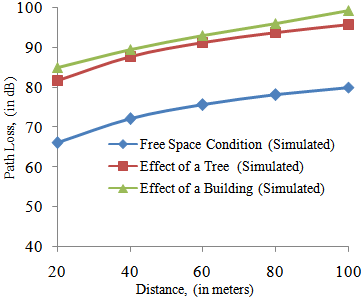Figure 9. Plots of Simulated Path Loss with Distance for the Three Models at Frequency of 2400 MHz
##### 4.1.2. Received Power Level in dB as a Function of Transmitting and Receiving Antennas Distance for the Models
From the result of the free space path loss, suggested tree path loss, and building path loss model shown in table 4, received power level for the three scenarios were obtained using the relationships: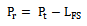(40)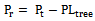(41)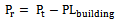(42)
We employed equation (40) under free space condition, equation (41) when the Lionet signal was obstructed by a single tree, and equation (42) when the Lionet signal was obstructed by a building.
Figure 10 shows that the received power level under free space condition is greater than that when the signal is obstructed by a single tree or a building. The results showed that the distance between the transmitting and receiving antennas and the received power level are inversely related.
Furthermore, the received power level under free space condition decreased from the highest power level of -47.07 dB to the lowest power level of -61.05 dB. Also, when the Lionet signal was obstructed by a single tree, the received power level decreased from the highest power level of -62.77 dB to the lowest power level of -76.75 dB. When the signal was obstructed by a building, the received power level dropped from the highest power level of -66.10 dB until the lowest power level of -80.28 dB. These showed that the received power level decreases as the distance between the transmitting and receiving antennas increases.
 Table 5. Results of the Simulated Received Power Level under Free Space Condition and under the effect of a Single Tree or a Building at Frequency of 2400 MHz
 TransmissionDistance(in meters) Received Power Level (in dB) Free Space Condition Effect of a Single Tree Effect of a Building 20 -47.07 -62.77 -66.10 40 -53.09 -68.79 -70.59 60 -56.61 -72.31 -74.16 80 -59.11 -74.81 -77.17 100 -61.05 -76.75 -80.28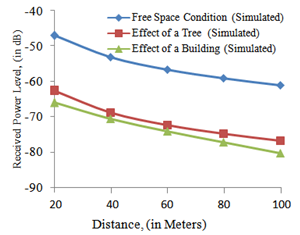Figure 10. Simulated Received Power Level with Distances for the Three Models at Frequency of 2400 MHz

#### 4.2. Field Measurements Results and Discussions

##### 4.2.1. Received Power Level in dB as a Function of Transmitting and Receiving Antennas Distance under Free Space, and Non Line-of-Sight Condition
Table 6 is the results from field measurements taken under free space condition and non line-of-sight condition (areas where the Lionet signal is obstructed by a single tree or a building). Also, their corresponding plots are figure 11.
 Table 6. Measured Received Power Level as a Function of Distance between the Transmitting and Receiving antennas under Free Space, and where the Lionet signal is obstructed by a Single Tree or a Building
 TransmissionDistances(in meters) Mean Received Power Level (in dB) Free Space Condition Effect of a Single Tree Effect of a Building 20 -51.28 -55.70 -59.00 40 -52.80 -58.20 -65.64 60 -53.88 -59.44 -66.24 80 -54.20 -60.56 -66.96 100 -57.56 -68.20 -69.88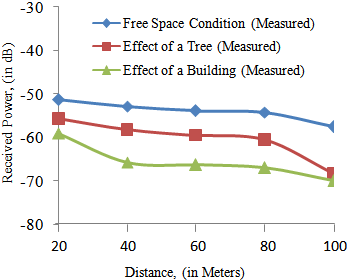Figure 11. Plots of the Measured Received Power Level with Distance for the Three Scenarios at Frequency of 2400 MHz
Figure 11 shows that the output result under the free space condition is higher due to no obstruction from trees or a building on the Lionet signal. This was attributed to the fact that under free space, the signal was assumed to be travelling under a clear line-of-sight. But in the presence of a tree or a building, part of the energy of the signal was absorbed by the tree canopy elements such as the leaves, branches, and construction materials used for the building etc.
Furthermore, the received power level under the free space condition decreased from the highest power level of -51.28 dB to the lowest power level of -54.20 dB. Also when the signal was obstructed by a single tree, the received power level decreased from the highest power level of -55.70 dB the lowest power level of -60.56 dB. When the signal was obstructed by a building, the received power level decreased from the highest level of -59.00 dB to the lowest power level of -66.96 dB. These indicated that the received power level under the considered three scenarios are distance dependent. Under the free space condition and when the signal was obstructed by a single tree, the received power level decreased gradually with the distance between 20 and 40 meters range. But when the signal was obstructed by a building a sharp decrease in the received power level was observed. Also the received power level seemed to decay faster with the distance between 80 and 100 meters range for the three conditions. But this was almost constant for the distance between 40 and 80 meters range for the three conditions.
##### 4.2.2. Path Loss in dB as a Function of Transmitting and Receiving Antennas Distance under Free Space, and Non Line-of-Sight Condition
From the measured received power level taken under free space and areas where the Lionet signal is obstructed by a single tree or a building, the measured path loss for the three scenarios shown in table 7 were obtained using the relationships: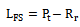(43)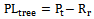(44)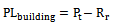(45)
The equation (43) was employed under free space condition, equation (44) when the Lionet signal was obstructed by a single tree, and equation (45) when the Lionet signal was obstructed by a building.
 Table 7. Results of the Measured Path loss under Free Space and the effect of a Single Tree or Building at Frequency of 2400 MHz
 TransmissionDistances(in meters) Mean Path Loss (in dB) Free Space Path Loss(PLFS) Tree Path Loss(PLtree) Building Path loss(PLbuilding) 20 70.28 74.70 78.00 40 71.80 77.20 84.64 60 72.88 78.44 85.24 80 73.20 79.56 85.96 100 76.56 87.20 88.88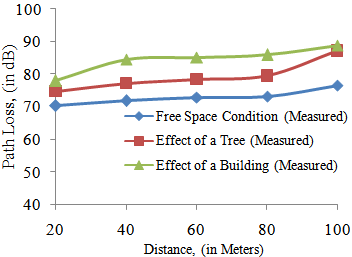Figure 12. Plots of the Measured Path Loss with Distances for the Three Scenarios at Frequency of 2400 MHz
Figure 12 shows that the path loss is higher when Lionet signal was either obstructed by a building or a tree as compared to when the signal was free from any form of obstruction. This was attributed to the fact that the propagation of radio signal through free space was not impeded by obstruction as compared when there were obstructed by trees and buildings along its propagation path. Also the path loss increased gradually with distance between 20 and 40 meters range, under free space condition and when the Lionet signal was obstructed by a single tree. The path loss increased sharply when the Lionet signal was obstructed by a building. The path loss seemed to rise faster with the distance between 80 and 100 meters range for the three conditions. But this was almost constant for the between 40 and 80 meters range for the three conditions.

#### 4.3. Comparison of the Simulated Results with the Field Measurement Results for the Three Scenarios

The simulated path loss results and the measured path loss results due to the obstruction of Lionet signal by a single tree and a building were compared using the simulated and measured free space path loss results as the reference point.Comparing the simulated free space path loss with that due to the effect of a single tree, the losses at different distances under consideration was 15.7dB. For that due to the effect of a building, we found the losses at different distances of consideration between 17.5 and 19.23 dB. Also, comparing the measured free space path loss with that due to the effect of a single tree, the losses at different distances under consideration were between 4.42 and 10.64 dB. For the case due to the effect of a building, the losses at different distances under consideration were between 7.72 and 12.84 dB. The result obtained for the scenario where the Lionet signal was obstructed by a single tree, agreed with the field measurement results obtained by Karlsson et al in which the path loss is between 1 and 16 dB at 3.1 and 5.8 GHz frequencies. Also, the results obtained due to the obstruction of the Lionet signal by a building conformed to result of building penetration loss reported by Durgin et al at an average value of 14 dB. This implies that the effects of trees and buildings on campus wireless networks are quite significant.

### 5. Conclusions

This work has studied the effects of the obstruction of trees and buildings on the Lionet wireless network. The study revealed that one of the problems that hinder efficient performance of the Lionet wireless network is the presence of trees and built structures. This resulted in poor signal reception, missing of data packets, delay in uploading and downloading of data, and fluctuation of signals. The mathematical models that described this physical problem were formulated, and simulated in computer program written in MATLAB. The simulation results showed reasonable agreement with experimental results for the studied three scenarios. The results showed that attenuation due to the presence of trees and buildings in the Lionet communication path is substantial. The results also showed that the Path loss increased, while the received power level decreased with the distance of separation between the transmitting and the receiving antennas, and vice versa. This study therefore recommends that the power level of the Lionet signal strength should be improved for efficient performance; more access points should be deployed at locations where obstructions are prevalent, and Lionet should possibly migrate from wireless to fibre optic network.

### References

  Robert K. Crane, Propagation Handbook for Wireless Communication System Design: CRC Press LLC, Boca Raton London New York Washington, D.C., 2003.  Robert E. Collin, Antennas and Radio Wave Propagation: McGraw Hill Inc, 1985.  Theodore S. Rappaport, Wireless Communication, Principles and Practice, 2nd ed., Pearson Education Inc. Singapore, 2000.  Y. S. Meng, Y. H. Lee, B. C. Ng, “Study of Propagation Loss Prediction in Forest Environment”, Progress in Electromagnetics Research B, Vol. 17, 117-133, 2009.  Tilahun Abebe, “Modeling of Scattering and Absorption by Phantom Tissue”, Addis Ababa University Addis Ababa, Ethiopia, 2010.  Sng Sin Hie, “Radio Channel Modeling For Mobile Ad Hoc Wireless Networks”, Naval Postgraduate School Monterey, California, 2004.  M. S. H. Al Salameh , Y. S. A. Al-Mashhadani, “Impact of a Concrete Room on the Performance of Cellular Telephone Communications for Low Bit Rate Applications”, ARPN Journal of Engineering and Applied Sciences, VOL. 6, 2011.  Y. S. Meng, Y. H. Lee, B. C. Ng, “Study of Propagation Loss Prediction in Forest Environment” Progress in Electromagnetics Research B, Vol. 17, 117-133, 2009.  R.K. Tewari, S. Swarup, and M. N. Roy, “Radio Wave Propagation through Rain Forests of India” IEEE Transactions on Antennas and Propagation, Vol.38, No.4, pp. 433-499, 1990.  Pon Lai Ly, Phoon Lai Ly, Tharek Abd. Rahman, Mohammed Khomeini Abu, “Investigation of Foliage Effects via Remote Data Logging at 5.8 GHz”, Wireless Communication Centre, Faculty of Electrical Engineering Universiti Teknologi Malaysia, 2010.  Oriuwa Richard Chukwudi, “The Effects of Vegetation on GSM Signal Propagation in Rural Areas”, M. Eng. Thesis, University of Nigeria Nsukka, Nigeria, 2008.  Michael J. Gans, Noach Amitay, Y. S. Yeh, T. C. Damen, Reinaldo A. Valenzuela, Choelhang Cheon, Jeho Lee, “ Measurements for Fixed Wireless Loops (FWL) in a Suburban Region with Foliage and Terrain Blockages”, IEEE Transactions on Wireless Communications, Vol.1, No.2, pp. 302- 310, 2002.  T. Sofos and P. Constantinou, “Propagation Model for Vegetation Effects in Terrestrial and Satellite Mobile Systems”, IEEE Transactions on Antennas and Propagation, Vol.52, No.7, 1917-1920, 2004.  S. Perras and L. Bouchard, “Fading Characteristics of RF Signals Due to Foliage in Frequency Bands from 2 to 60 GHz”, The 5th International Symposium on Wireless Personal Multimedia Communications, pp. 267- 271, 2002.  J. Goldhirsh and W. J. Vogel, Roadside Tree Attenuation Measurements at UHF for Land Mobile Satellite Systems, IEEE Transactions on Antennas and Propagation, Vol.35, No.5, pp. 589-596, 1987.  A. Karlsson, R.E. Schuh, C. Bergljung, P. Karlsson, and N. Lowendahl, “The Influence of Trees on Radio Channel at Frequencies of 3 and 5 GHz”, IEEE VTS 54th Vehicular Technology Conference, pp. 2008-2012, 2001.  Yvo L. C. de Jong, Matti H. A. J. Herben, “A Tree-Scattering Model for Improved Propagation Prediction in Urban Microcells”, IEEE Transaction on Vehicular Technology, Vol. 53, No. 2, 2004.  Peter Johannesson, “Wave Propagationthrough Vegetation at 3.1 GHz and 5.8 GHz”, Department of Electroscience, Lund Institute of Technology Sweden, 2001.  Kiyoshi Honda, “Interactions between Electromagnetic Wave and Targets” Space Technology Applications and Research Program School of Advanced Technologies Asian Institute of Technology.  Kamal Sarabandi, “Electromagnetic scattering from vegetation canopies” PhD Thesis, University of Michigan, 1989.  ITU-R, Recommendation ITU-R P.841, “Conversion of Annual Statistics to Worst-Month Statistics”, International Telecommunication Union, Geneva, 1994.  G. A. 1. Van Dooren, “A deterministic approach to the modelling of Electromagnetic wave propagation in urban environments", Ph.D. Thesis, Eindhoven University of Technology, Netherlands, 1994.  S. Stavrou and S.R. Saunders. “Review of constitutive parameters of building materials”, Centre for Communication Systems Research University of Surrey, United Kingdom.  Karlsson A., R.E. Schuh, C. Bergljung, P. Karlsson, and N. Lowendahl, “The Influence of Trees on Radio Channel at Frequencies of 3 and 5 GHz”, IEEE VTS 5th Vehicular Technology Conference, pp. 2008-2012, 2001.  Durgin G., T.S. Rappaport, and H. Xu., “Measurements and Model for Radio Pathloss and Penetration Loss in and Around Homes and Trees at 5.85 GHz”, IEEE Transactions on Communication, Vol. 46, No.11, pp. 1484-1496, 1998.